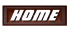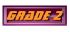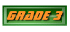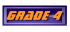Report Errors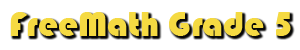Place Value
1A Value of Underlined Digit - up to Billions
1B Number Words & Expanded Form to Billions
1C Math Vocabulary - "Periods"
1D Compare Numbers "< >" to tens of Millions
1E Ordering Numbers Least to Greatest
1F Problem Solving
Place Value: Decimals
2A Decimals - Standard Form
2B Decimals - Word Form
2C Decimals - Value of Underlined Digit
2D Decimals - Compare < > =
2E Decimals - Ordering Least to Greatest
2F Equivalent Decimals
2G Decimals - Problem Solving
Whole Numbers & Decimals
3C Subtract Decimals
3D Rounding Whole Numbers to Millions Place
3E Name Place to which Number is Rounded
3F Round Decimals to Underlined Digit
3G Estimate Sums (Round to 100,000)
3H Estimate Differences (Round to 100,000)
3I Addition: 6 and 7 Digits
3J Find Missing Digits in Addition Problem
3K Subtraction: 6 and 7 Digits
3L Subtraction: Find Missing Digits
3M Decimals: Problem Solving
Multiplication
4A Multiply 5 & 6 Digit by 1-Digit Number
4B Multiply by 2-digit Number
4C Estimate Products
4D Problem Solving
5A Multiply Decimals and Whole Numbers
5B Multiplication Patterns
5C Multiply Decimals by Decimals
5D Exponents
Division
6A Estimate Quotients
6B 1-Digit Divisors
6C Problem Solving
7A Estimate Quotients - Compatible Numbers
7B Divide by 2-Digit Divisors
7C Dividing Decimals
7D Divide Whole Numbers (decimal quotient)
7E Problem solving
Graphs and Interpreting Data
8A Vocabulary
8B Range, Mode, Median, Mean
8C Vocabulary
8D Making a Bar Graph
8E Frequency Table
8F Coordinate Grid
8G Make a Line Graph
9A Vocabulary
9B Histogram
9C Stem & Leaf Plot
9D Circle Graph
Number Theory
10A Divisibility Rules
10B Prime and Composite Numbers
10C Common Factors - GCF
Fractions
10D Write the Fraction Shown by the Model
10E Equivalent Fractions
10F Simplify Fractions
10G Mixed Numbers
11A Least Common Multiple - LCM
11B Least Common Denominator - LCD
11C Equivalent Fractions
11D Compare Fractions
11E Order Fractions
12A Add & Subtract Like  Fractions
12B Add & Subtract Unlike Fractions
12D Subtract Fractions Using LCD
12E Relate Fractions & Decimals
12F Problem Solving
13B Subtract Mixed Numbers
13C Subtract Fractions with Renaming
14A Multiply Whole Number by Fraction
14B Multiply a Fraction by a Fraction
14C Multiply Fractions & Mixed Numbers
14D Multiply Mixed Numbers
15A Reciprocals
15B Divide Whole Numbers by Fractions
15C Divide Fractions
15D Problem Solving (multiply fractions)
15E Problem Solving (divide fractions)
Measurement: Customary Units
16A Length
16B Capacity and Weight
16C Time
Measurement: Metric Units
17A Length
17B Capacity & Mass
17C Temperature
Algebra
18B Expressions (Multiply & Divide)
18C Order of Operations
18D Functions
18E Graphing a Function
19B Equations (Subtract)
19C Equations (Multiply)
19D Equations (Divide)
Geometry
20A Lines
20B Measure Angles
20C Classify Angles
20D Triangles
21A Circles
21B Congruent and Similar Figures
21C Transformations
21D Tessellations
Perimeter & Area
22A Find Perimeter
22B Area of Squares & Rectangles
22C Area of Parallelograms
22D Area of Triangles
Surface Area and Volume
23A Nets and 3-Dimensional Figures
23B Surface Area
23C Estimate and Find Volume

Ratio
25A Ratios
25B Ratios and Proportions
25C Equivalent Ratios: use cross products
25D Equivalent Ratios: find the missing number
25E Scale Drawings
Probability
26A Probability
26B Outcomes
26C Combinations
Percent
27A Understanding Percent
27B Fractions, Decimals & Percents
27C Find Percent of a Number
27D Circle Graphs